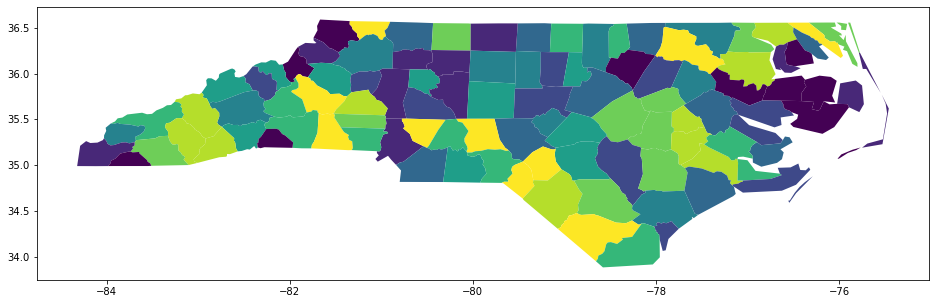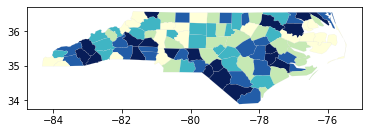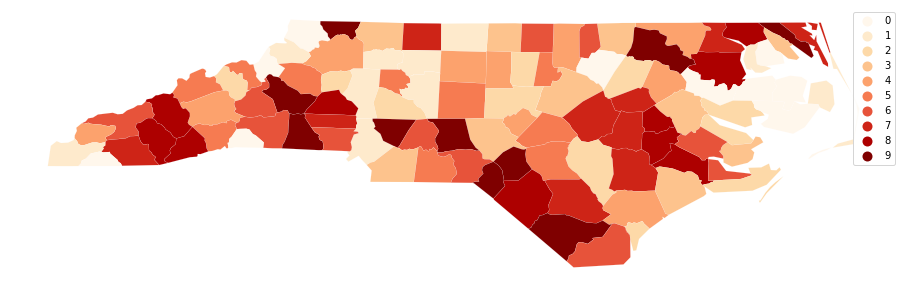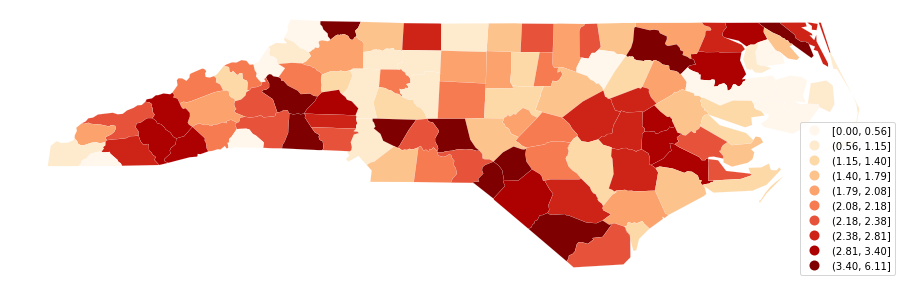# Choropleth¶

mapclassify is intended to be used with visualizaiton packages to handle the actual rendering of the choropleth maps defined on its classifiers. In this notebook, we explore some examples of how this is done. The notebook also includes an example that combines mapclassify with ipywidgets to allow for the interactive exploration of the choice of:

• classification method
• number of classes
• colormap
In :
import libpysal
import geopandas as gpd
import mapclassify

In :
mapclassify.__version__

Out:
'2.3.0'

The example in this notebook use data on sudden death infant syndrome for counties in North Carolina which is a built-in dataset available through libpysal. We use libpysal to obtain the path to the shapefile and then use geopandas to create a geodataframe from the shapefile:

In :
libpysal.examples.explain('sids2')

sids2
=====

North Carolina county SIDS death counts and rates
-------------------------------------------------

* sids2.dbf: attribute data. (k=18)
* sids2.shp: Polygon shapefile. (n=100)
* sids2.shx: spatial index.
* sids2.gal: spatial weights in GAL format.

Source: Cressie, Noel (1993). Statistics for Spatial Data. New York, Wiley, pp. 386-389. Rates computed.
Updated URL: https://geodacenter.github.io/data-and-lab/sids2/


In :
pth = libpysal.examples.get_path('sids2.shp')

In :
gdf.head()

Out:
AREA PERIMETER CNTY_ CNTY_ID NAME FIPS FIPSNO CRESS_ID BIR74 SID74 NWBIR74 BIR79 SID79 NWBIR79 SIDR74 SIDR79 NWR74 NWR79 geometry
0 0.114 1.442 1825 1825 Ashe 37009 37009 5 1091.0 1.0 10.0 1364.0 0.0 19.0 0.916590 0.000000 9.165903 13.929619 POLYGON ((-81.47276 36.23436, -81.54084 36.272...
1 0.061 1.231 1827 1827 Alleghany 37005 37005 3 487.0 0.0 10.0 542.0 3.0 12.0 0.000000 5.535055 20.533881 22.140221 POLYGON ((-81.23989 36.36536, -81.24069 36.379...
2 0.143 1.630 1828 1828 Surry 37171 37171 86 3188.0 5.0 208.0 3616.0 6.0 260.0 1.568381 1.659292 65.244668 71.902655 POLYGON ((-80.45634 36.24256, -80.47639 36.254...
3 0.070 2.968 1831 1831 Currituck 37053 37053 27 508.0 1.0 123.0 830.0 2.0 145.0 1.968504 2.409639 242.125984 174.698795 MULTIPOLYGON (((-76.00897 36.31960, -76.01735 ...
4 0.153 2.206 1832 1832 Northampton 37131 37131 66 1421.0 9.0 1066.0 1606.0 3.0 1197.0 6.333568 1.867995 750.175932 745.330012 POLYGON ((-77.21767 36.24098, -77.23461 36.214...

Once created, the geodataframe has a plot method that can be called to create our first choropleth map. We will specify the column to classify and plot as SIDR79: SIDS death rate per 1,000 births (1979-84). The classification scheme is set to Quantiles, and the number of classes set to k=10 (declies):

In :
gdf.plot(column='SIDR79', scheme='Quantiles', k=10, figsize=(16, 9))

Out:
<matplotlib.axes._subplots.AxesSubplot at 0x7f3b05b6fd10>We can peak under the hood a bit and recreate the classification object that was used in the previous choropleth:

In :
q10 = mapclassify.Quantiles(gdf.SIDR79,k=10)
q10

Out:
Quantiles

Interval     Count
--------------------
[0.00, 0.56] |    10
(0.56, 1.15] |    10
(1.15, 1.40] |    10
(1.40, 1.79] |    10
(1.79, 2.08] |    10
(2.08, 2.18] |    10
(2.18, 2.38] |    10
(2.38, 2.81] |    10
(2.81, 3.40] |    10
(3.40, 6.11] |    10

For quick, exploratory work, the classifier object has its own plot method that takes a geodataframe as an argument:

In :
_ = q10.plot(gdf)Back to working directly with the dataframe, we can toggle on the legend:

In :
import matplotlib.pyplot as plt
f, ax = plt.subplots(1, figsize=(16, 9))
gdf.assign(cl=q10.yb).plot(column='cl', categorical=True, \
k=10, cmap='OrRd', linewidth=0.1, ax=ax, \
edgecolor='white', legend=True)
ax.set_axis_off()
plt.show()Here we see the 10 classes, but without more specific information on the legend, the user has to know that 0 is the first declile and 9 the 10th. We also do not know the values that define these classes.

We can rectify this as follows:

In :
q10.get_legend_classes()

Out:
['[0.00, 0.56]',
'(0.56, 1.15]',
'(1.15, 1.40]',
'(1.40, 1.79]',
'(1.79, 2.08]',
'(2.08, 2.18]',
'(2.18, 2.38]',
'(2.38, 2.81]',
'(2.81, 3.40]',
'(3.40, 6.11]']
In :
mapping = dict([(i,s) for i,s in enumerate(q10.get_legend_classes())])

In :
mapping

Out:
{0: '[0.00, 0.56]',
1: '(0.56, 1.15]',
2: '(1.15, 1.40]',
3: '(1.40, 1.79]',
4: '(1.79, 2.08]',
5: '(2.08, 2.18]',
6: '(2.18, 2.38]',
7: '(2.38, 2.81]',
8: '(2.81, 3.40]',
9: '(3.40, 6.11]'}
In :
def replace_legend_items(legend, mapping):
for txt in legend.texts:
for k,v in mapping.items():
if txt.get_text() == str(k):
txt.set_text(v)

import matplotlib.pyplot as plt
f, ax = plt.subplots(1, figsize=(16, 9))
gdf.assign(cl=q10.yb).plot(column='cl', categorical=True, \
k=10, cmap='OrRd', linewidth=0.1, ax=ax, \
edgecolor='white', legend=True,
legend_kwds={'loc': 'lower right'})
ax.set_axis_off()
replace_legend_items(ax.get_legend(), mapping)
plt.show()## Interactive Exploration of Choropleth Classification¶

Next, we develop a small application that relies on mapclassify together with palettable and ipywidgets to explore the choice of:

• classification method
• number of classes
• colormap
In :
from palettable import colorbrewer
sequential = colorbrewer.COLOR_MAPS['Sequential']
diverging = colorbrewer.COLOR_MAPS['Diverging']
qualitative = colorbrewer.COLOR_MAPS['Qualitative']

In :
from ipywidgets import interact, Dropdown, RadioButtons, IntSlider, VBox, HBox, FloatSlider, Button, Label

k_classifiers = {
'equal_interval': mapclassify.EqualInterval,
'fisher_jenks': mapclassify.FisherJenks,
'jenks_caspall': mapclassify.JenksCaspall,
'jenks_caspall_forced': mapclassify.JenksCaspallForced,
'maximum_breaks': mapclassify.MaximumBreaks,
'natural_breaks': mapclassify.NaturalBreaks,
'quantiles': mapclassify.Quantiles,
}

def k_values(ctype, cmap):
k = list(colorbrewer.COLOR_MAPS[ctype][cmap].keys())
return list(map(int, k))

def update_map(method='quantiles', k=5, cmap='Blues'):
classifier = k_classifiers[method](gdf.SIDR79, k=k)
mapping = dict([(i,s) for i,s in enumerate(classifier.get_legend_classes())])
#print(classifier)
f, ax = plt.subplots(1, figsize=(16, 9))
gdf.assign(cl=classifier.yb).plot(column='cl', categorical=True, \
k=k, cmap=cmap, linewidth=0.1, ax=ax, \
edgecolor='grey', legend=True, \
legend_kwds={'loc': 'lower right'})
ax.set_axis_off()
ax.set_title("SIDR79")
replace_legend_items(ax.get_legend(), mapping)

plt.show()

bindings = {'Sequential': range(3,9+1),
'Diverging': range(3,11+1),
'Qualitative': range(3,12+1)}

cmap_bindings = {'Sequential': list(sequential.keys()),
'Diverging': list(diverging.keys()),
'Qualitative': list(qualitative.keys())}

class_val = Dropdown(options=bindings[data_type.value], value=5)
cmap_val = Dropdown(options=cmap_bindings[data_type.value])

def type_change(change):
class_val.options = bindings[change['new']]
cmap_val.options = cmap_bindings[change['new']]

def cmap_change(change):
cmap=change['new']
ctype = data_type.value
k = k_values(ctype, cmap)
class_val.options = k

data_type.observe(type_change, names=['value'])
cmap_val.observe(cmap_change, names=['value'])

from ipywidgets import Output, Tab
out = Output()
t = Tab()
t.children = [out]
#t

# In this case, the interact function must be defined after the conditions stated above...
# therefore, the k now depends on the radio button

with out:
interact(update_map, method=list(k_classifiers.keys()), cmap=cmap_val, k = class_val)

display(VBox([data_type, out]))


Changing the type of colormap (sequential, diverging, qualitative) will update the options for the available color maps (cmap). Changing any of the values using the dropdowns will update the classification and the resulting choropleth map.

It is important to note that the example variable is best portrayed with the sequential colormaps. The other two types of colormaps are included for demonstration purposes only.